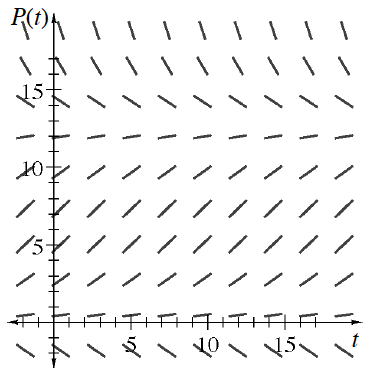### Home > CALC3RD > Chapter Ch7 > Lesson 7.3.6 > Problem7-165

7-165.

Stingray populations grow based on the differential equation below, where $P$ is the population (in thousands) and $t$ is time in years. Use the graph of the slope field at right to complete parts (a) through (c) below.

$\frac { d P } { d t } = 0.026P(12.5 − P)$

1. If there are $3000$ stingrays for time $t = 0$, sketch a curve representing the population of stingrays.

The starting point of the curve is $\left(0, 3000\right)$.

2. What if the original population when $t = 0$ is $10,000$ stingrays? Draw this population curve and decide if its rate of growth is the same or different as that in part (a).

The starting point of the curve is $\left(0, 10,000\right)$.

3. What if the original population when $t = 0$ is $17,000$ stingrays? Draw this population curve and decide if its rate of growth is the same or different as those in parts (a) and (b).

Based on parts (a) and (b), you should have noticed that both curves approached the same value over time.
The starting point of this curve is $(0, 17000)$. Do you think it will approach the same threshold?